# Green space

(diff) ← Older revision | Latest revision (diff) | Newer revision → (diff)
A topological spaceon which harmonic and superharmonic functions (cf. Harmonic function; Subharmonic function) are defined and for which a Green function exists (for the Dirichlet problem in the class of harmonic functions) or, which amounts to the same thing, for which there exists a non-constant superharmonic function. More exactly, letbe an-space, i.e. a connected separable topological space in which: 1) each pointhas an open neighbourhood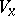homeomorphic to some open setof a Euclidean space(or of its Aleksandrov compactification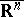); and 2) the images of any non-empty intersection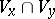(under the two homeomorphisms toor) of two neighbourhoods in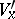and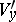are isometric, and are conformally equivalent if. Harmonic and superharmonic functions on an-spaceare locally defined by passing to the images. If, in addition, there exists a non-constant positive superharmonic function onor, which amounts to the same thing, a positive potential,is known as a Green space. Thus, the Euclidean space, its compactification() and Riemann surfaces are all-spaces. Here,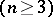and Riemann surfaces of hyperbolic type (cf. Riemann surfaces, classification of) are Green spaces, while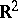and Riemann surfaces of parabolic type are not. Any domain in a Green spaceis again a Green space.
A harmonic spacewith a positive potential on it can also be regarded as a generalization of a Green space in the framework of axiomatic potential theory (cf. Potential theory, abstract).